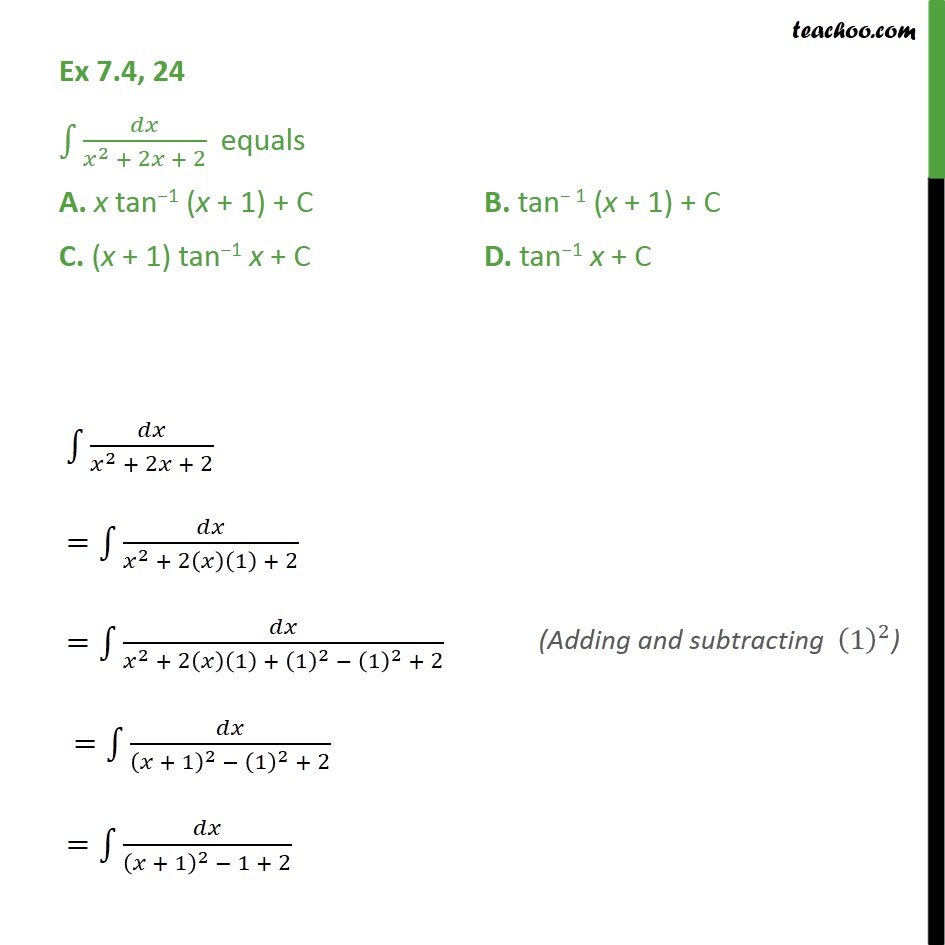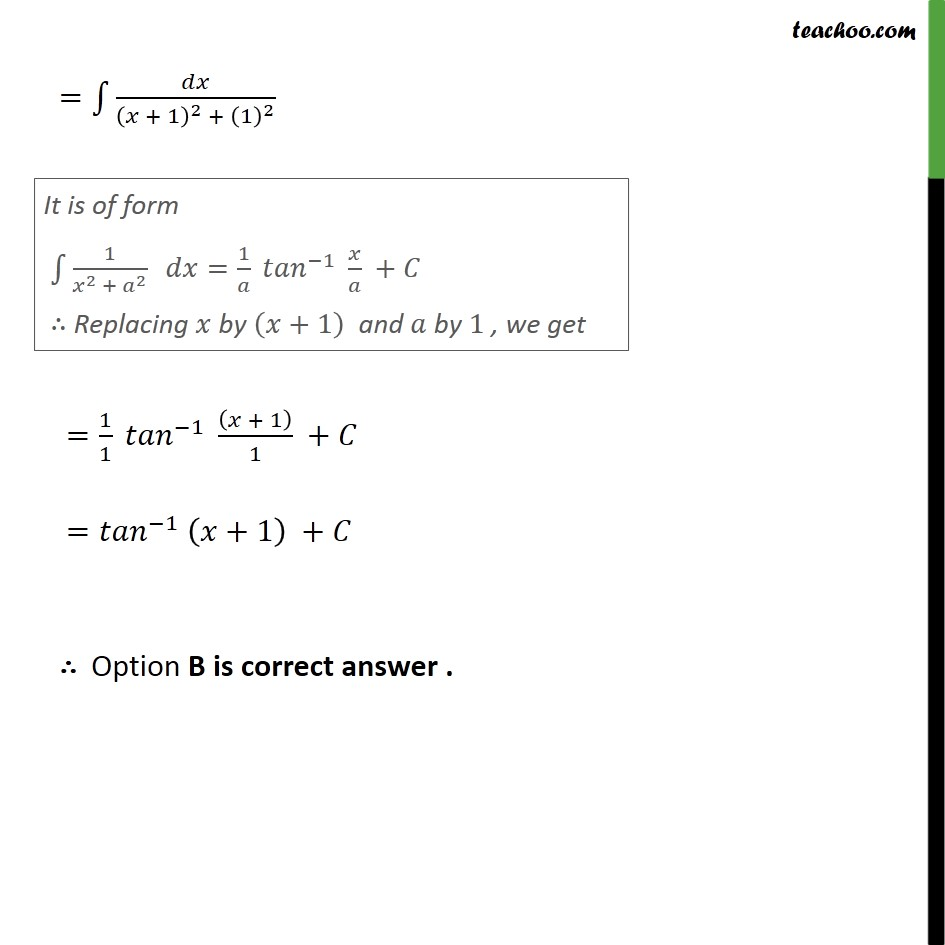1. Chapter 7 Class 12 Integrals (Term 2)
2. Serial order wise
3. Ex 7.4

Transcript

Ex 7.4, 24 2 + 2 + 2 equals A. x tan 1 (x + 1) + C B. tan 1 (x + 1) + C C. (x + 1) tan 1 x + C D. tan 1 x + C 2 + 2 + 2 = 2 + 2 1 + 2 = 2 + 2 1 + 1 2 1 2 + 2 = + 1 2 1 2 + 2 = + 1 2 1 + 2 = + 1 2 + 1 2 = 1 1 1 + 1 1 + = 1 +1 + Option B is correct answer .

Ex 7.4